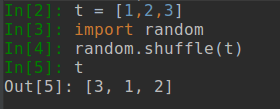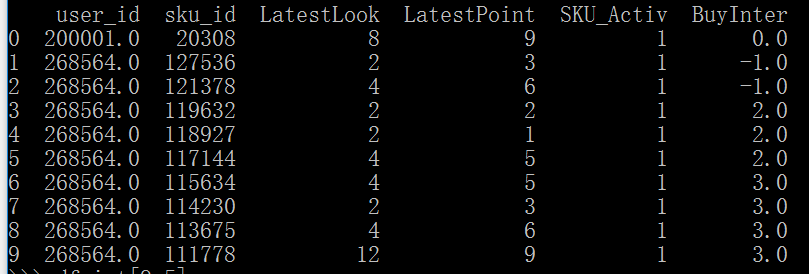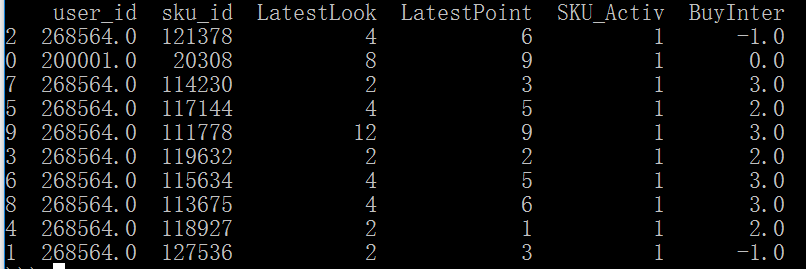• ## python随机打乱列表

千次阅读 2019-04-18 22:36:38
python随机打乱列表 python自带的random库用于实现与随机处理有关的功能 python数据打乱列表的方法 t = [1,2,3] random.shuffle(t)
python随机打乱列表
python自带的random库用于实现与随机处理有关的功能
python数据打乱列表的方法
t = [1,2,3]
random.shuffle(t)展开全文• ## python随机打乱样本

千次阅读 2018-06-19 17:55:17
可以将列表随机打乱[python] view plain copyli=range(20) random.shuffle(li)
可以将列表随机打乱df=range(20)  1.python自带的：return random.shuffle(li) 2.from sklearn.utils import shuffle  df = shuffle(df)  在Python里面，使用Pandas里面的DataFrame来存放数据的时候想要把数据集进行shuffle会许多的方法，本文介绍两种比较常用而且简单的方法。应用情景：我们有下面以个DataFrame我们可以看到BuyInter的数值是按照0,-1,-1,2,2,2,3,3,3,3这样排列的，我们希望不保持这个次序，但是同时列属性又不能改变，即如下效果：实现方法：最简单的方法就是采用pandas中自带的 sample这个方法。假设df是这个DataFramedf.sample(frac=1)  这样对可以对df进行shuffle。其中参数frac是要返回的比例，比如df中有10行数据，我只想返回其中的30%,那么frac=0.3。有时候，我们可能需要打混后数据集的index（索引）还是按照正常的排序。我们只需要这样操作df.sample(frac=1).reset_index(drop=True)  另外，numpy库中也有进行shuffle的方法（不建议）df.iloc[np.random.permutation(len(df))]
展开全文• 现在有一个list:[1,2,3,4,5,6]，我需要把这个list在输出的时候，是以一种随机打乱的形式输出。 专业点的术语：将一个容器中的数据每次随机逐个遍历一遍。 注意：不是生成一个随机的list集。 环境： Python 3.6 解决...
场景：
现在有一个list:[1,2,3,4,5,6]，我需要把这个list在输出的时候，是以一种随机打乱的形式输出。
专业点的术语：将一个容器中的数据每次随机逐个遍历一遍。
注意：不是生成一个随机的list集。
环境：
Python 3.6
解决方案：
方案一：
有人可能会通过Random内置函数，来间接实现想要的结果。但是这种方式，太原始，也不够优雅，而且有种重复造轮子的嫌疑。这里我就不贴我自己通过random实现的效果了。
方案二：
Random中有一个random.shuffle()方法提供了完美的解决方案。代码如下：
x = [1,2,3,4,5,6]
random.shuffle(x)
print(x)
输出结果：
第一次输出内容：[6, 5, 1, 3, 2, 4]
第二次输出内容：[6, 1, 3, 5, 2, 4]
第三次输出内容：[5, 3, 1, 2, 4, 6]
从结果我们可以看出，输出是完全随机的，代码量就两行，不需要random，不需要for循环。
源码解读：
此部分原文链接：Python中打乱列表顺序 random.shuffle()的使用方法
def shuffle(self, x, random=None):
"""Shuffle list x in place, and return None.
原位打乱列表，不生成新的列表。

Optional argument random is a 0-argument
function returning a random float in [0.0, 1.0);
if it is the default None,
the standard random.random will be used.
可选参数random是一个从0到参数的函数，返回[0.0,1.0)中的随机浮点；
如果random是缺省值None，则将使用标准的random.random()。
"""

if random is None:
randbelow = self._randbelow
for i in reversed(range(1, len(x))):
# pick an element in x[:i+1] with which to exchange x[i]
j = randbelow(i + 1)
x[i], x[j] = x[j], x[i]
else:
_int = int
for i in reversed(range(1, len(x))):
# pick an element in x[:i+1] with which to exchange x[i]
j = _int(random() * (i + 1))
x[i], x[j] = x[j], x[i]


注意 ：
从代码的注释，我们看到random.shuffle()是对原list做修改，如果需要保留原list，请注意这个细节。
本文首发于BigYoung小站


展开全文• 不会生成新的列表，只是将原列表的次序打乱 # shuffle()使用样例 import random x = [i for i in range(10)] print(x) random.shuffle(x) print(x) 源码及注释（个人翻译注释） def shuffle(self, x, random=None...
• 现在有个需求需要将列表的次序打乱，或者也可以这样理解： 【需求】将一个容器中的数据每次随机逐个遍历一遍。 random.shuffle()方法提供了完美的解决方案。 不会生成新的列表，只是将原列表的次序打乱 # shuffle()...
之前自己一直使用random中 randint生成随机数以及使用for将列表中的数据遍历一次。
现在有个需求需要将列表的次序打乱，或者也可以这样理解：
【需求】将一个容器中的数据每次随机逐个遍历一遍。
random.shuffle()方法提供了完美的解决方案。
不会生成新的列表，只是将原列表的次序打乱
# shuffle()使用样例
import random

x = [i for i in range(10)]
print(x)
random.shuffle(x)
print(x)

源码及注释（个人翻译注释）
def shuffle(self, x, random=None):
"""Shuffle list x in place, and return None.
原位打乱列表，不生成新的列表。

Optional argument random is a 0-argument
function returning a random float in [0.0, 1.0);
if it is the default None,
the standard random.random will be used.
可选参数random是一个从0到参数的函数，返回[0.0,1.0)中的随机浮点；
如果random是缺省值None，则将使用标准的random.random()。
"""

if random is None:
randbelow = self._randbelow
for i in reversed(range(1, len(x))):
# pick an element in x[:i+1] with which to exchange x[i]
j = randbelow(i + 1)
x[i], x[j] = x[j], x[i]
else:
_int = int
for i in reversed(range(1, len(x))):
# pick an element in x[:i+1] with which to exchange x[i]
j = _int(random() * (i + 1))
x[i], x[j] = x[j], x[i]

random 中其他的方法
class Random(_random.Random):

## -------------------- integer methods  -------------------
def randrange(self, start, stop=None, step=1, _int=int):

def randint(self, a, b):

def _randbelow(self, n, int=int, maxsize=1 << BPF, type=type,
Method=_MethodType, BuiltinMethod=_BuiltinMethodType):

## -------------------- sequence methods  -------------------
def choice(self, seq):

def shuffle(self, x, random=None):

def sample(self, population, k):

def choices(self, population, weights=None, *, cum_weights=None, k=1):

## -------------------- uniform distribution -------------------
def uniform(self, a, b):

## -------------------- triangular --------------------
def triangular(self, low=0.0, high=1.0, mode=None):

## -------------------- normal distribution --------------------
def normalvariate(self, mu, sigma):

## -------------------- lognormal distribution --------------------
def lognormvariate(self, mu, sigma):

## -------------------- exponential distribution --------------------
def expovariate(self, lambd):

## -------------------- von Mises distribution --------------------
def vonmisesvariate(self, mu, kappa):

## -------------------- gamma distribution --------------------
def gammavariate(self, alpha, beta):

## -------------------- Gauss (faster alternative) --------------------
def gauss(self, mu, sigma):

def betavariate(self, alpha, beta):

## -------------------- Pareto --------------------
def paretovariate(self, alpha):

## -------------------- Weibull --------------------
def weibullvariate(self, alpha, beta):



展开全文• 方案一： import numpy as np array = np.arange(0, 10, 1) print("原来数据顺序:") print(array) state = np.random.get_state() np.random.shuffle(array) ...print("打乱原顺序，新的随机数据:") print(ar...
• from random import shuffle def shuffle_str(s): # 将字符串转换成列表 ... # 调用random模块的shuffle函数打乱列表 shuffle(str_list) # 将列表转字符串 return ''.join(str_list) ...
• list_0 = ['A', 'B', 'C', 'D', 'E', 'F', 'G'] # 列表不能设置中间变量（等同于原变量） list_1 = [] while len(list_0) > 0: # 随机生成索引 x = random.randint(0, len(list_0) - 1) list_1.append(list...
• java可以用Collections.shuffle(List)来实现，python怎么实现呢？ python要利用random模块的shuffle方法 代码如下： import random x = [i for i in range(10)] print(x) random.shuffle(x) print(x) ...
• 数组 采用numpy.random.get_state() , numpy.random.set_state() list列表 随机种子random.seed: zip方式：
• //随机打乱列表顺序 验证码功能： 转载于:https://www.cnblogs.com/jefy/p/9303745.html
• 用来把一个列表中元素的顺序随机打乱，用法如下 #!/usr/bin/python3 import random list = [20, 16, 10, 5]; random.shuffle(list) print ("随机排序列表 : ", list) random.shuffle(list) ...
• help(random.shuffle) ...可以将列表随机打乱 [python] view plain copy li=range(20) random.shuffle(li)  ########################################## help(random.random)
• 数组 采用numpy.random.get_state() , numpy.random.set_state() list列表 随机种子random.seed: zip方式：
• @python实现随机时间段与时间点打乱存储并由csv生成excel表 一. 时间片段的随机获取 二. 时间点的随机获取 三. 列表内数据打乱存储 四. list转为csv 五. csv转为excel表展示 时间片段的随机获取 在某一段时间内，...csv
• 一个随机划分图片数据集的方法。...处理过程：读取文件列表，将列表打乱，截取列表一部分 import os import random import shutil def get_imlist(path): return [os.path.join(path, f) for f in os.listdir(...数据集划分 深度学习
• 前言   随机数可以用于数学，游戏，安全等领域中，还经常被嵌入到算法中，用以提高算法效率，并提高程序的安全性。...现在MP3的随机列表就是用的伪随机，把要播放的歌曲打乱顺序，生成一个随机
• random模块 导入 import random 随机整数 包括最大值，不可加步长 random.randint(最小值，最大值) ...随机取多个，返回结果是列表 random.sample(列表，取几个) 打乱顺序 用于洗牌，会改变列...
• import random #随机抽取一个元素，String类型也可以随机返回一个字符 ...#随机打乱列表random.shuffle()是没有返回值的，直接更新列表 m_random = [1, 2, 3, 4, 5, 6, 7, 8, 9, 10] random.shuffle(m_random) ...
• Python标准库中的random函数，可以生成随机浮点数、整数、字符串，甚至帮助你随机选择列表序列中的一个元素，打乱一组数据等。1、random模块方法说明random.random()函数是这个模块中最常用的方法了，它会生成一个...
• Python标准库中的random函数，可以生成随机浮点数、整数、字符串，甚至帮助你随机选择列表序列中的一个元素，打乱一组数据等。 1、random模块方法说明 random.random()函数是这个模块中最常用的方法了，它会生成一...
• 这篇文章主要介绍了python中的随机函数...Python标准库中的random函数，可以生成随机浮点数、整数、字符串，甚至帮助你随机选择列表序列中的一个元素，打乱一组数据等。 二、random模块重要函数 1 )、random() 返...random
• 一、random模块简介Python标准库中的random函数，可以生成随机浮点数、整数、字符串，甚至帮助你随机选择列表序列中的一个元素，打乱一组数据等。 二、random模块重要函数1 )、random() 返回0<=n<1之间的随机...
• Python标准库中的random函数，可以生成随机浮点数、整数、字符串，甚至帮助你随机选择列表序列中的一个元素，打乱一组数据等。 二、random模块重要函数 1 )、random() 返回0 2 )、choice(seq) ...
• 方法一： np.random.shuffle （无返回值，直接将原数组重新随机...方法二： 返回一个打乱的index列表，可将其用于多个数组的按同一随机顺序进行排序 shuffle_ix = np.random.permutation(np.arange(len(train_data)))数据结构 机器学习 数据挖掘...

# python随机打乱列表python 订阅